IF(周末是晴天吗？和朋友去郊游,去书店看书)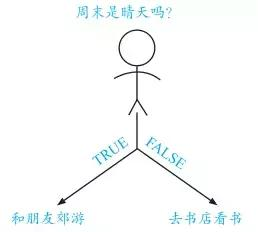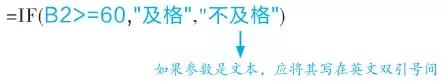Excel收到你输入的公式后，便会按你指定的评定标准去评定成绩的等次。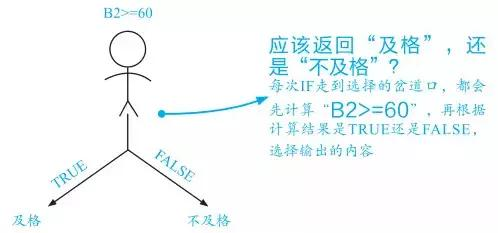“如果”的后面是要判断的条件，“那么”的后面是条件成立时返回的结果，“否则”的后面是条件不成立时返回的结果。只有按这个规则去设置IF的各个参数，Excel才会明白你的意图。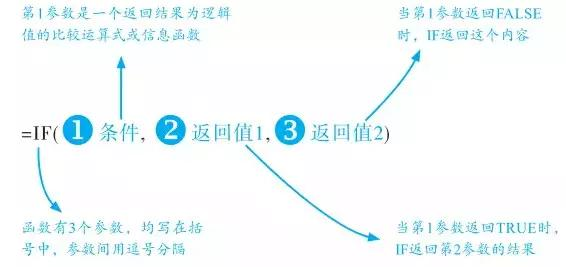IF共有3个参数，每个参数扮演不同的角色，只有参数设置正确，Excel才会明白你的意图。

1、IF+AND函数多条件判断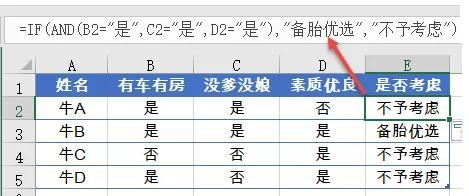=IF(AND(B2=\"是\",C2=\"是\",D2=\"是\"),\"备胎优选\",\"不予考虑\")

AND函数的作用是依次判断多个条件，如果多个条件的判断结果都是TRUE，AND函数最终返回TRUE，否则就返回 最终返回TRUE，否则就返回FALSE。

=IF(OR(B2=\"是\",C2=\"是\",D2=\"是\"),\"备胎优选\",\"不予考虑\")

OR函数的作用也是依次判断多个条件，只要多个条件的判断结果有一个是TRUE，OR函数就返回TRUE。

2、IF+IF 多区间判断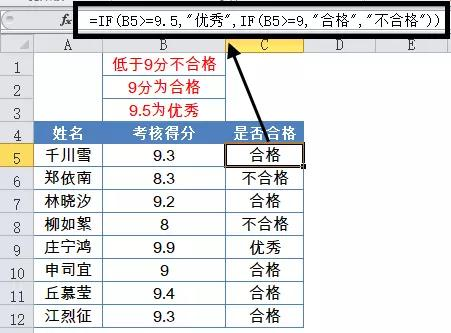=IF(B5>=9.5,\"优秀\",IF(B5>=9,\"合格\",\"不合格\"))